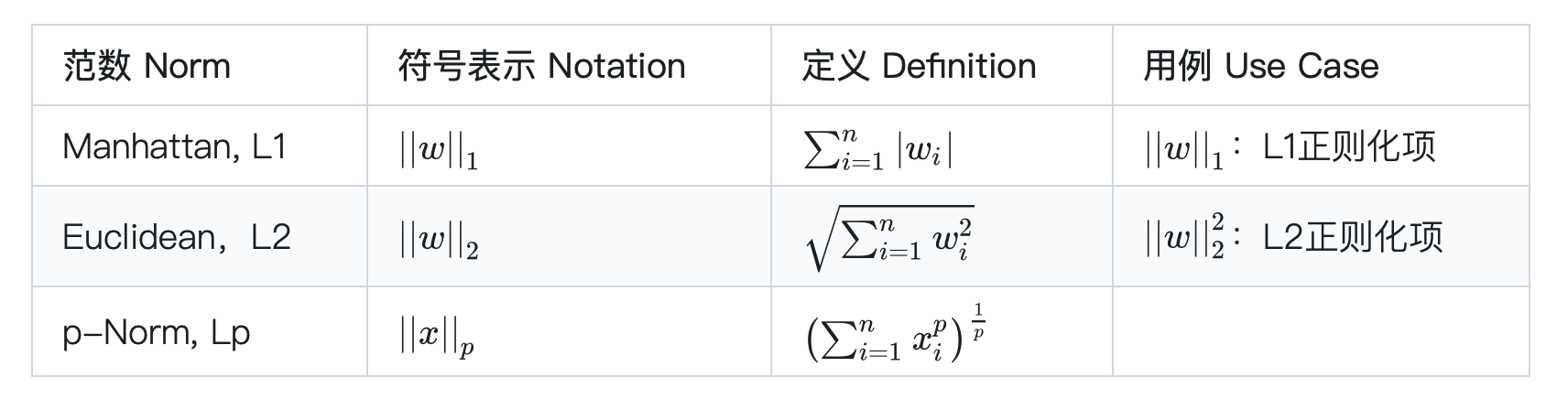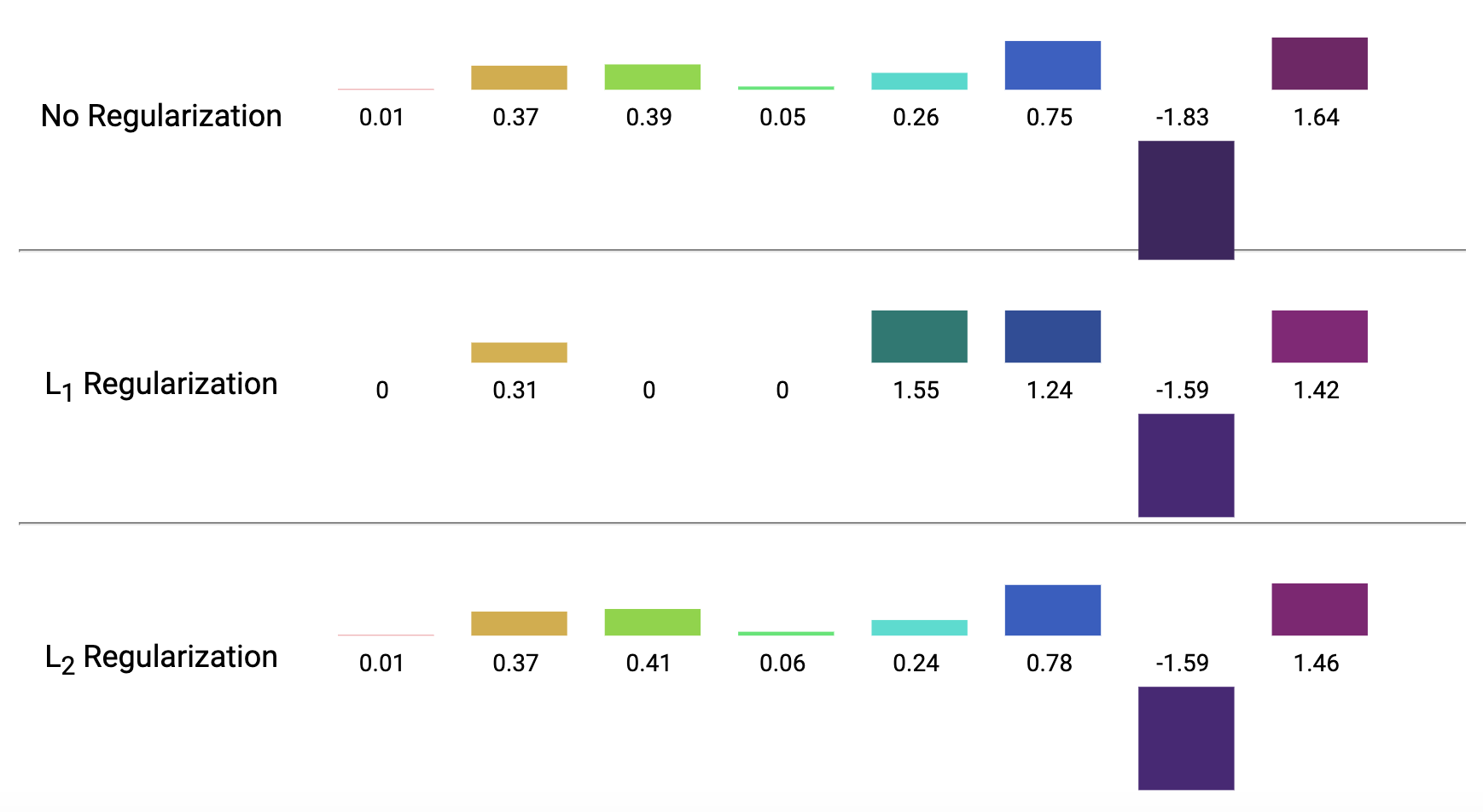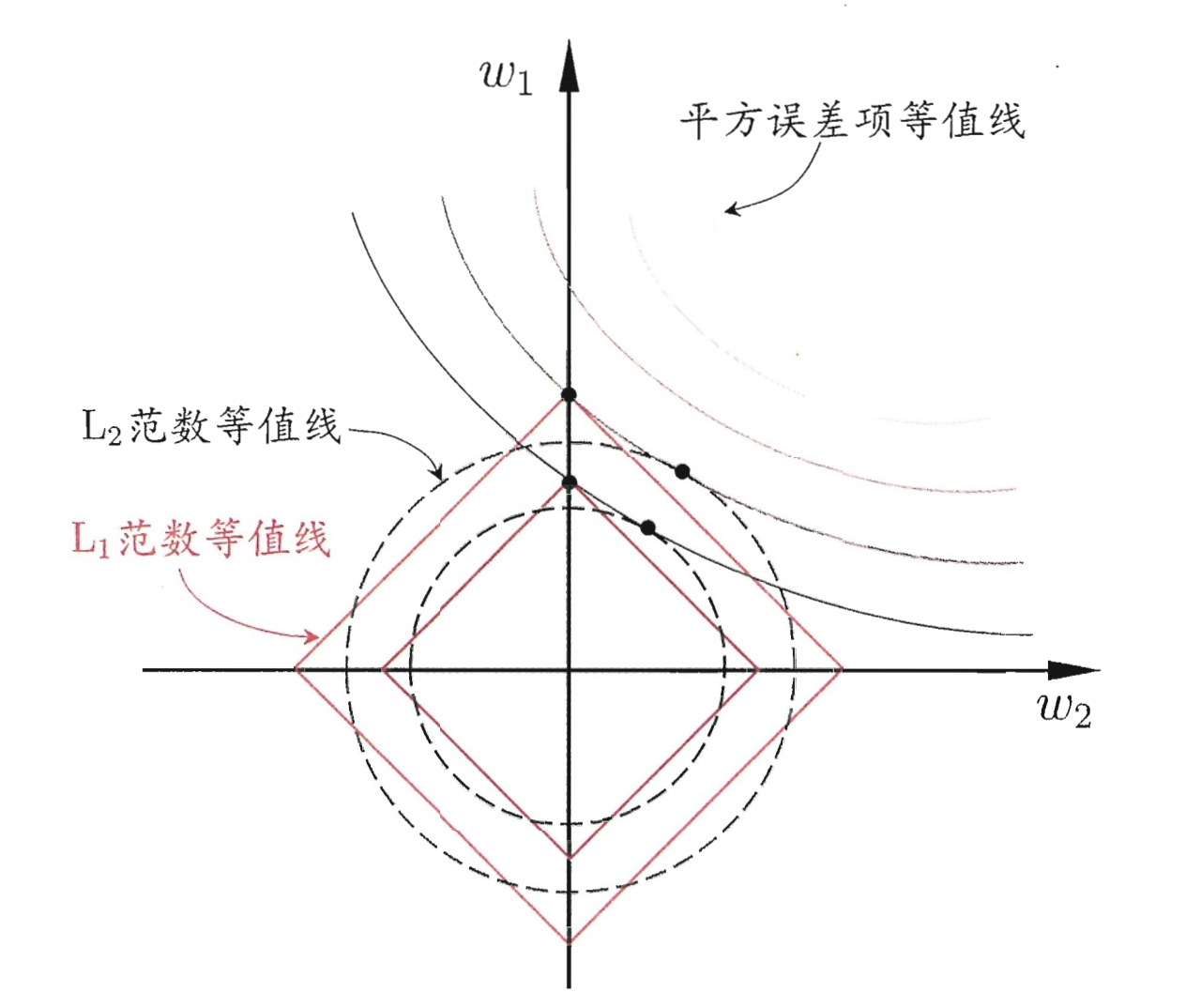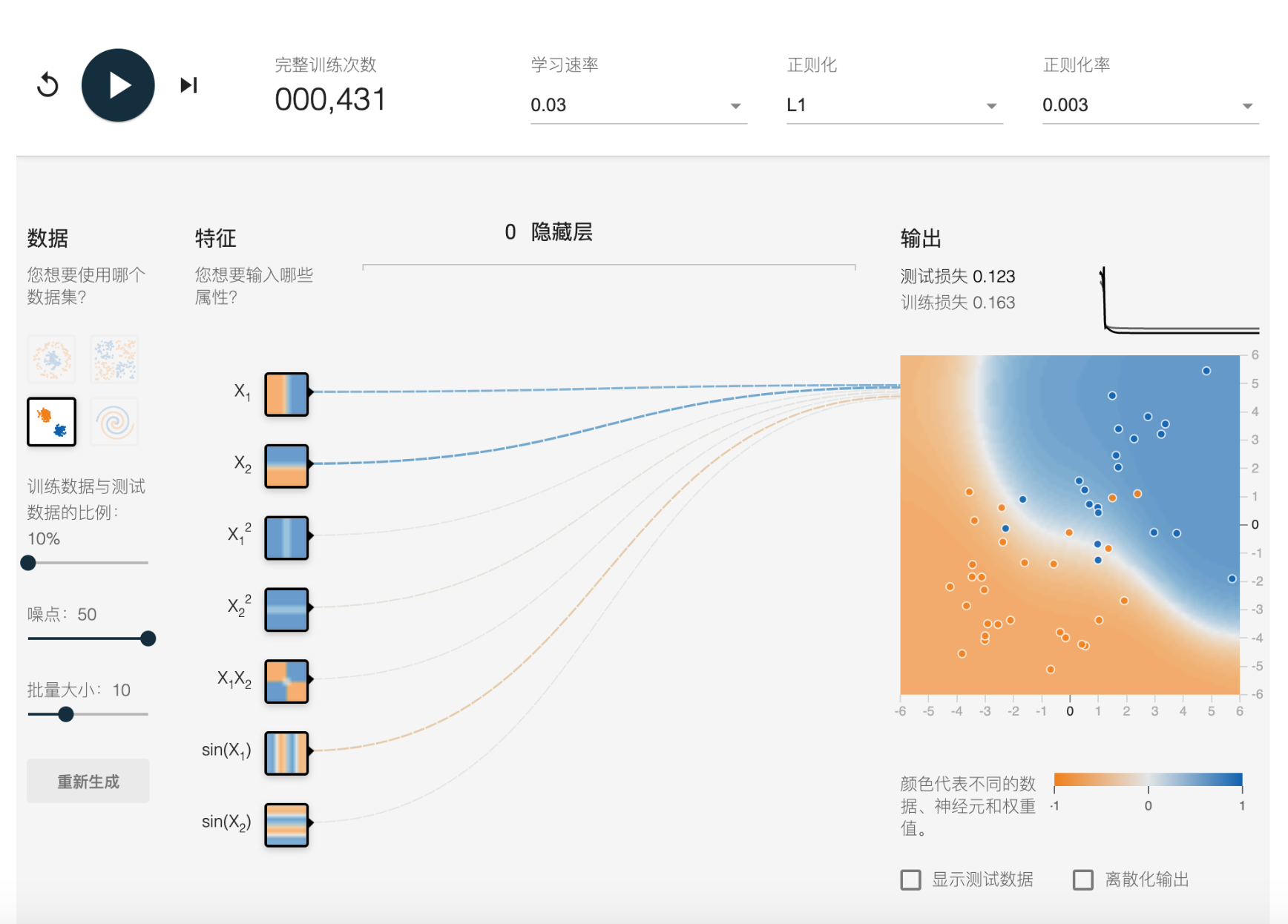# 正则化：防止模型过拟合

## 岭回归 Ridge Regression#

\begin{aligned} L(\boldsymbol{w}) &= \frac{1}{2}\sum_{i=1}^m(f_\boldsymbol{w}(\boldsymbol{x}^{(i)}) - y^{(i)})^2 \\ &= \frac{1}{2}\sum_{i=1}^m(\boldsymbol{w}^\top\boldsymbol{x}^{(i)} - y^{(i)})^2 \end{aligned}

$L(\boldsymbol{w}) = \frac{1}{2}\left(\sum_{i=1}^m(\boldsymbol{w}^\top\boldsymbol{x}^{(i)} - y^{(i)})^2 + \lambda\sum_{j=1}^nw_j^2\right)$

$\frac \partial {\partial w_j}L(w) = \sum_{i=1}^m(\boldsymbol{w}^\top\boldsymbol{x}^{(i)} - y^{(i)})x_{j}^{(i)} + \lambda w_j$

## Lasso回归 Lasso Regression#

$L(\boldsymbol{w}) = \frac{1}{2}\left(\sum_{i=1}^m(\boldsymbol{w}^\top\boldsymbol{x} - y)^2 + \lambda\sum_{j=1}^n|w_j|\right)$

## 一般正则项### 稀疏解与L1正则化#L1和L2正则化的区别 来源：Google DevelopersL1正则化比L2正则化更容易使得参数为零 来源：《机器学习》

## 正则化系数#

$minimize\left(Loss(Data|Model)) + \lambda \times complexity(Model) \right)$

• 如果正则化系数过大，模型可能比较简单，但是有欠拟合的风险。模型可能没有学到训练数据中的一些特性，预测时也可能不准确。
• 如果正则化系数过小，模型会比较复杂，但是有过拟合的风险。模型努力学习训练数据的各类特性，但泛化预测能力可能不高。
• 理想的正则化系数可以让模型有很好的泛化能力，不过，正则化系数一般与训练数据、业务场景等具体问题相联系，因此需要通过调参找到一个较优的选项。

## 练习和巩固#TensorFlow Playground

1. Andrew Ng：CS229 Lecture Notes
2. Ian Goodfellow and Yoshua Bengio and Aaron Courville: 《Deep Learning》
3. 周志华：《机器学习》
4. https://stanford.edu/~shervine/teaching/cs-229/cheatsheet-machine-learning-tips-and-tricks